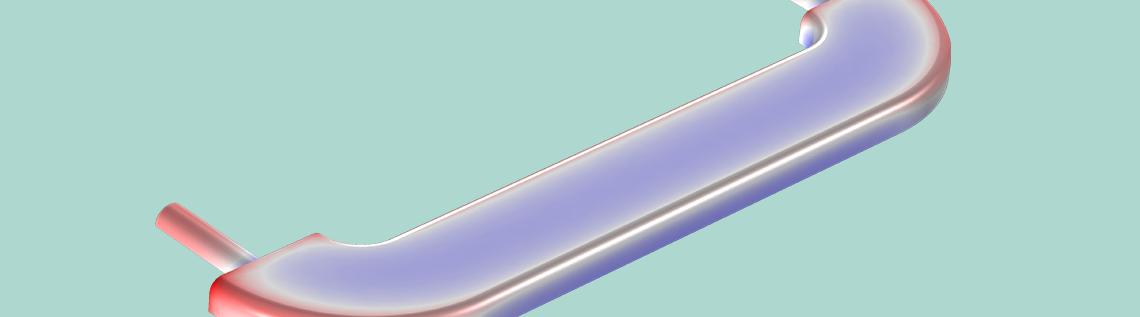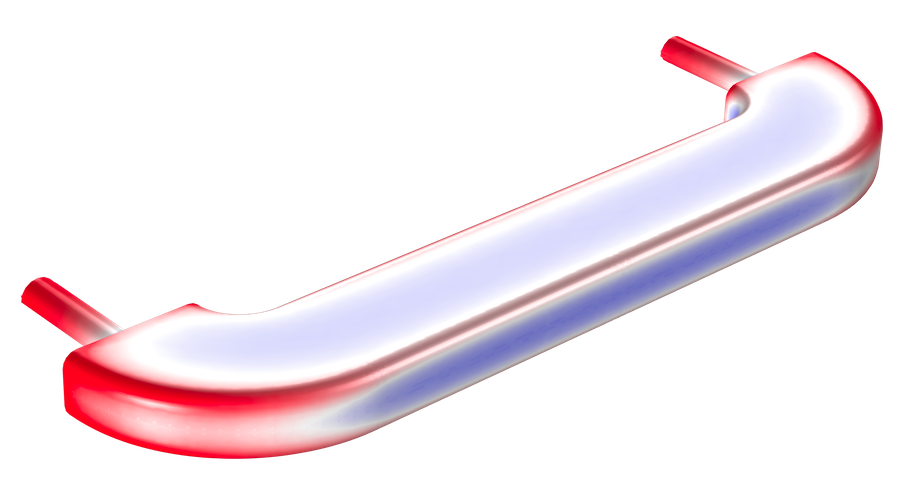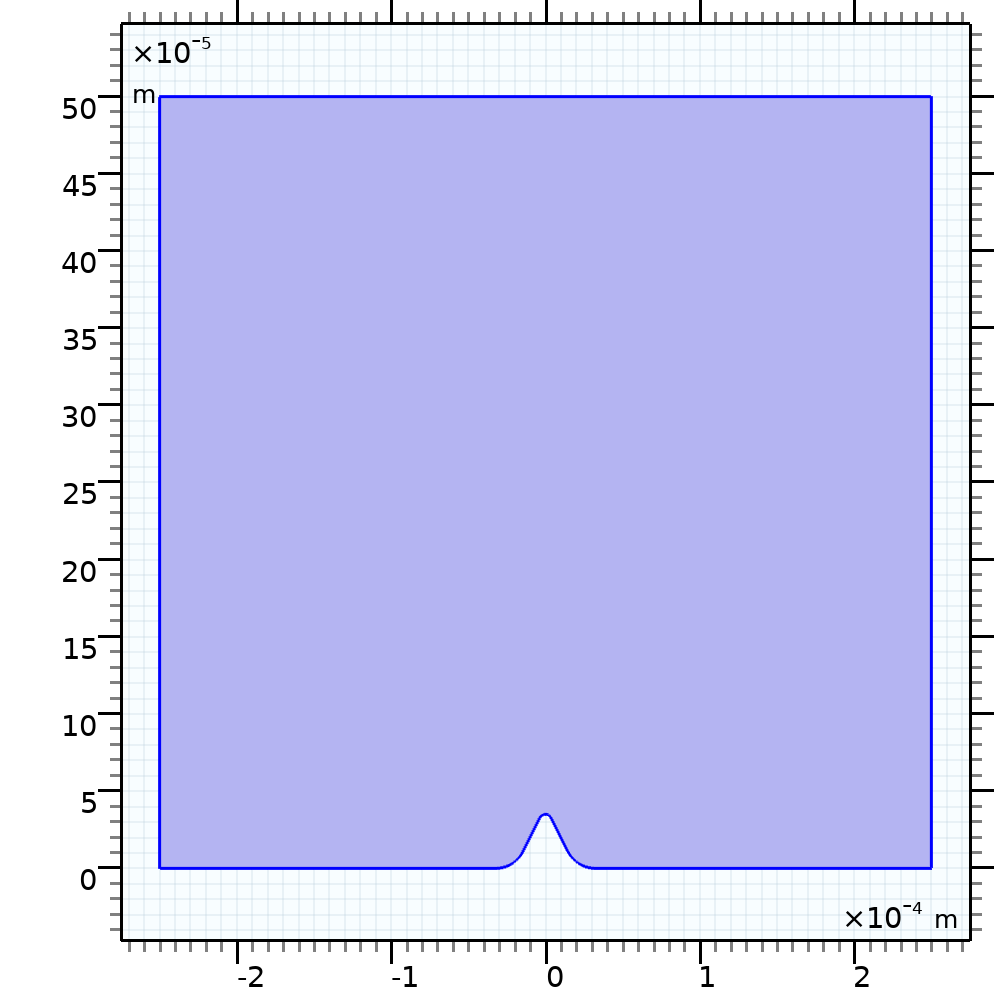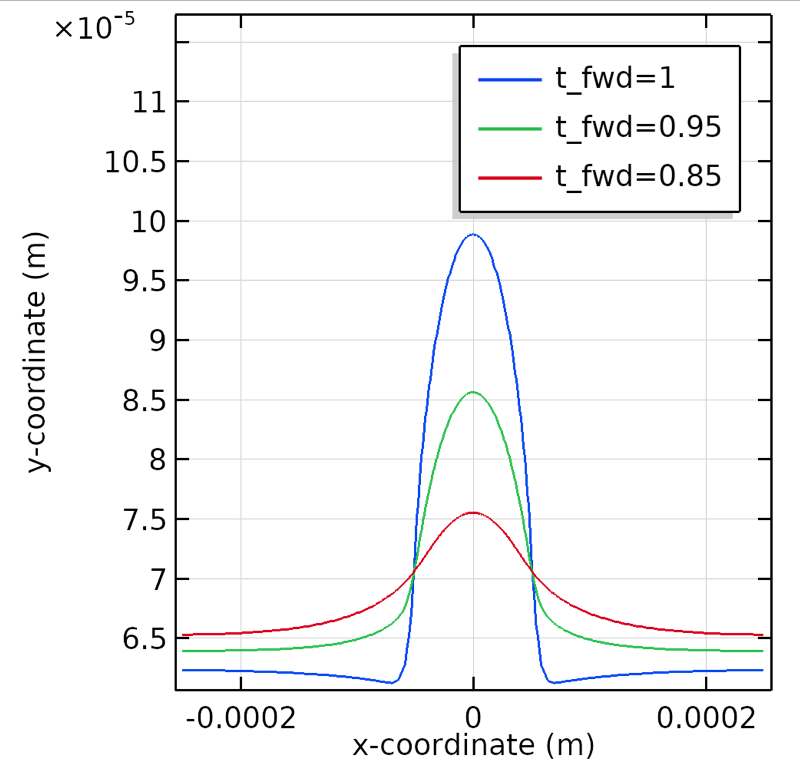# 电镀法的艺术和科学

2018年 11月 13日

### 什么是反向脉冲电镀？RPP 由正向占空比（t_{fwd} ）和反向占空比（t_{rev} ）组成，在正向占空比（t_{fwd} ）下，施加阴极电流，进行金属沉积（电镀），在反向占空比（t_{rev} ）下，电镀电流变为反向，进行金属离子溶解（退镀）。在每个方向（正向和反向），占空比定义为电镀/溶解时间与施加电流总时间的比值。占空比的平均电流密度由下式给出：

i_{avg}= i_{fwd}\times t_{fwd}+ i_{rev}\times t_{rev}\:\:\:\:\:(1)

i_{fwd}= \frac{i_{avg}-i_{rev}\times t_{rev}}{t_{fwd}}\:\:\:\:\:(2)

### 量化电流分布

Wa=\frac{\kappa}{\ell}\cdot\left(\frac{d\eta}{di}\right)\:\:\:\:\:(3)

Wa=\frac{\kappa}{ell}\cdot\left(\frac{\beta}{i}\right)\:\:\:\:\:(4)

### 使用 COMSOL Multiphysics® 为 RPP 建模

“案例下载”中的反向脉冲电镀模型利用二次电流分布 接口来分析活化过电位（反应动力学）和一次电流分布效应（几何效应和电解质电导率）。\sigma_l\nabla\cdot\phi_l=i_l\:\:\:\:\:(5)

\Sigma\, i_{loc}=i_{total}\:\:\:\:\:(6)

i_{loc}=i_0\left(exp\left(\frac
{\alpha_aF\eta}
{RT}\right)-exp\left(-\frac{\alpha_cF\eta}{RT}\right)\right)\:\:\:\:\:(7)

vn_{\textrm{bnd}}= \frac{M_{\textrm{Cu}} * R_{\textrm{Cu}}}{\rho_{\textrm{Cu}
}}=- \frac{M_{\textrm{Cu}} }{\rho_{\textrm{Cu}}}*\frac{\nu_{\textrm{Cu}}*i_{loc}}{nF}\:\:\:\:\:(8)### 反向脉冲如何提高电镀工艺水平？### 结束语

• 与直流电镀工艺相比，RPP 获得了更平滑的表面轮廓
• RPP 脉冲宽度的选择可以显著改变工作电极上的电流分布
• 通过调整正向与反向占空比的比率，可以获得更平滑的光洁度

### 后续操作

#### 评论 (1)

##### 留言##### 小灵 詹
2019-05-05

Hello, I have read your blog of reverse pulse plating model. It is a great job you have done. Considering the reverse pulse current applied on the electrode, you investigated the effect of duty circle on the plating. I have a question to ask, as the factors of RPP influencing the plating process includes not only duty circle, but also the frequency of the reverse pulse current. So, with this model, how you are going to simulate the plating condition with different frequency of the reverse pulse current, such as 1 Hz, 10 Hz. Thanks!